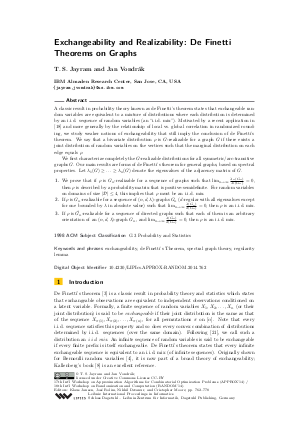Document# Exchangeability and Realizability: De Finetti Theorems on Graphs

### Authors T. S. Jayram, Jan Vondrák## File

LIPIcs.APPROX-RANDOM.2014.762.pdf
• Filesize: 492 kB
• 17 pages

## Cite As

T. S. Jayram and Jan Vondrák. Exchangeability and Realizability: De Finetti Theorems on Graphs. In Approximation, Randomization, and Combinatorial Optimization. Algorithms and Techniques (APPROX/RANDOM 2014). Leibniz International Proceedings in Informatics (LIPIcs), Volume 28, pp. 762-778, Schloss Dagstuhl - Leibniz-Zentrum für Informatik (2014)
https://doi.org/10.4230/LIPIcs.APPROX-RANDOM.2014.762

## Abstract

A classic result in probability theory known as de Finetti's theorem states that exchangeable random variables are equivalent to a mixture of distributions where each distribution is determined by an i.i.d. sequence of random variables (an "i.i.d. mix"). Motivated by a recent application and more generally by the relationship of local vs. global correlation in randomized rounding, we study weaker notions of exchangeability that still imply the conclusion of de Finetti's theorem. We say that a bivariate distribution rho is G-realizable for a graph G if there exists a joint distribution of random variables on the vertices such that the marginal distribution on each edge equals rho. We first characterize completely the G-realizable distributions for all symmetric/arc-transitive graphs G. Our main results are forms of de Finetti's theorem for general graphs, based on spectral properties. Let lambda_1(G) >= ... >= lambda_n(G) denote the eigenvalues of the adjacency matrix of G. 1. We prove that if rho is G_n-realizable for a sequence of graphs such that lambda_n(G_n) / lambda_1(G_n) tends to 0, then rho is described by a probability matrix that is positive-semidefinite. For random variables on domains of size |D| <= 4, this implies that rho must be an i.i.d. mix. 2. If rho is G_n-realizable for a sequence of (n,d,lambda)-graphs G_n (d-regular with all eigenvalues except for one bounded by lambda in absolute value) such that lambda(G_n) / d(G_n) tends to 0, then rho is an i.i.d. mix. 3. If rho is G_n-realizable for a sequence of directed graphs such that each of them is an arbitrary orientation of an (n,d,lambda)-graph G_n, and lambda(G_n) / d(G_n) tends to 0, then rho is an i.i.d. mix.
##### Keywords
• exchangeability
• de Finetti’s Theorem
• spectral graph theory
• regularity lemma

## Metrics

• Access Statistics
• Total Accesses (updated on a weekly basis)
0